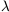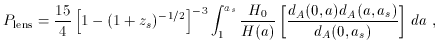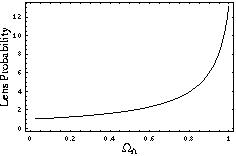3.4 Gravitational lensing

The volume of space back to a specified redshift, given by (44), depends sensitively on. Consequently, counting the apparent density of observed objects, whose actual density per cubic Mpc is assumed to be known, provides a potential test for the cosmological constant [112, 113, 114, 3] Like tests of distance vs. redshift, a significant problem for such methods is the luminosity evolution of whatever objects one might attempt to count. A modern attempt to circumvent this difficulty is to use the statistics of gravitational lensing of distant galaxies; the hope is that the number of condensed objects which can act as lenses is less sensitive to evolution than the number of visible objects.

In a spatially flat universe, the probability of a source at redshift zs being lensed, relative to the fiducial (M = 1,= 0) case, is given by(53)

where as = 1 / (1 + zs).Figure 9. Gravitational lens probabilities in a flat universe withM += 1, relative toM = 1,= 0, for a source at z = 2.

As shown in Figure (9), the probability rises dramatically asis increased to unity as we keepfixed. Thus, the absence of a large number of such lenses would imply an upper limit on.

Analysis of lensing statistics is complicated by uncertainties in evolution, extinction, and biases in the lens discovery procedure. It has been argued [115, 116] that the existing data allow us to place an upper limit of< 0.7 in a flat universe. However, other groups [117, 118] have claimed that the current data actually favor a nonzero cosmological constant. The near future will bring larger, more objective surveys, which should allow these ambiguities to be resolved. Other manifestations of lensing can also be used to constrain, including statistics of giant arcs , deep weak-lensing surveys , and lensing in the Hubble Deep Field .